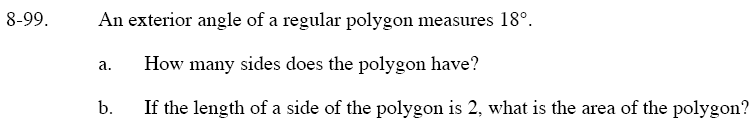Home > GC > Chapter 8 > Lesson 8.3.2 > Problem8-99

8-99.
1. An exterior angle of a regular polygon measures 18°. Homework Help ✎

1. How many sides does the polygon have?

2. If the length of a side of the polygon is 2, what is the area of the polygon?Remember that the sum of all exterior angles is equal to 360°.

Refer to the Math Notes box in Lesson 8.3.1, which gives a step-by-step example illustrating how to solve this type of problem.